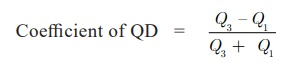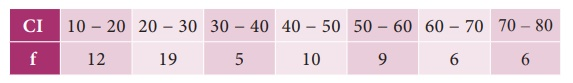Home | | Business Maths 11th std | Quartile Deviation

# Quartile Deviation

(i) Relative measures for QD (ii) Computation of Quartile Deviation

Quartile Deviation

Quartile Deviation is defined as QD = Â˝ (Q3 â€“ Q1) It may also be called as semi- inter quartile.

where Q1 and Q3 are the first and third quartiles of the distribution respectively and Q3 â€“ Q1 is called as inter quartile range.

## (i) Relative measures for QD

Quartile deviation is an absolute measure of dispersion. The relative measure corresponding to this measure, called the coefficient of quartile deviation is calculated as follows:Coefficient of quartile deviation can be used to compare the degree of variation in different distributions.

## (ii) Computation of Quartile Deviation

The process of computing quartile deviation is very simple since we just have to compute the values of the upper and lower quartiles that is Q3 and Q1 respectively.

Example 8.16

Calculate the value of quartile deviation and its coefficient from the following dataSolution :

Marks are arranged in ascending orderHence coefficient of QD = 0.455

Example 8.17

Compute coefficient of quartile deviation from the following dataSolution :Example 8.18

Compute Quartile deviation from the following dataSolution :Tags : Measures of dispersion , 11th Business Mathematics and Statistics(EMS) : Chapter 8 : Descriptive statistics and probability
Study Material, Lecturing Notes, Assignment, Reference, Wiki description explanation, brief detail
11th Business Mathematics and Statistics(EMS) : Chapter 8 : Descriptive statistics and probability : Quartile Deviation | Measures of dispersion